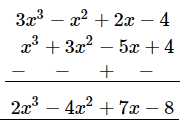# Subtract:

Question:

Subtract:
x3 + 3x2 − 5x + 4 from 3x3 − x2 + 2x − 4

Solution:

On arranging the terms of the given expressions in the descending powers of $x$ and subtracting column-wise: